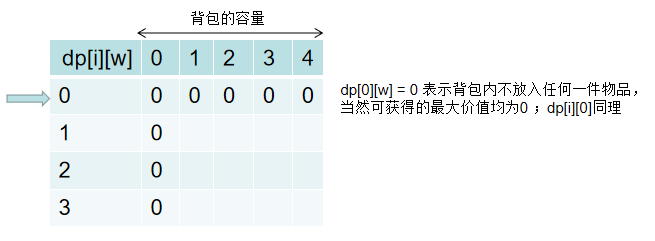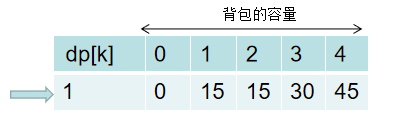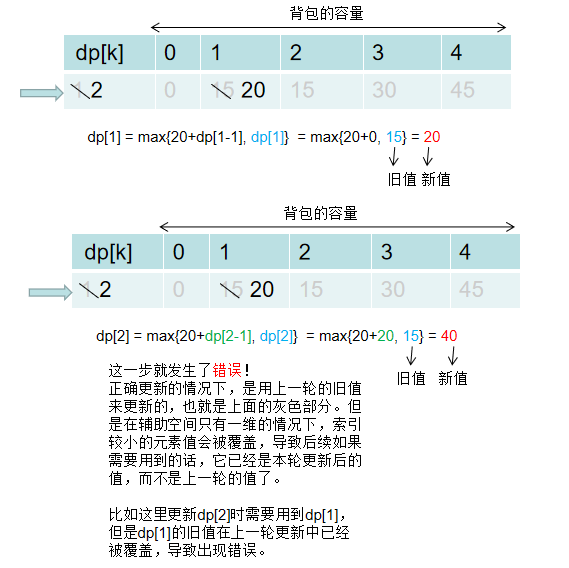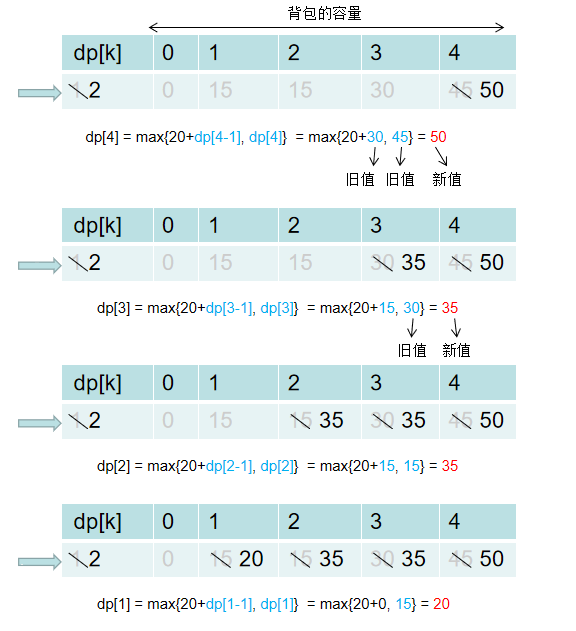01���������������������������,动态规划之01背包问题

01背包问题

01背包问题之另一种风格的描述：1. 吉他行2. 音响行3. 笔记本电脑行4. 等等，再增加一件商品将如何变化呢？3磅容量的最大价值为2000美元！再加上iPhone价值2000美元，总价值为4000美元。新的最大价值诞生了！dp[i][k] 的结果取两者的较大值，即：

dp[i][k] = max(value[i] + dp[i-1][k-weight[i]], dp[i-1][k])

public class BeiBao01 {        public int maxValue(int[] weight, int[] value, int W) {      //这里假定传入的weight和values数组长度总是一致的        int n = weight.length;        if (n == 0) return 0;​        int[][] dp = new int[n + 1][W + 1];        for (int i = 1; i = 1; k--) {                int valueWith_i = (k - weight[i] >= 0) ? (dp[k - weight[i]] + value[i]) : 0;                int valueWithout_i = dp[k];                dp[k] = Math.max(valueWith_i, valueWithout_i);          }      }        return dp[W];  }public int maxValue(int[] weight, int[] value, int W) {        int n = weight.length;        if (n == 0) return 0;​        int[] dp = new int[W + 1];        for (int i = 0; i < n; i++) {          //只要确保 k>=weight[i] 即可，而不是 k>=1，从而减少遍历的次数            for (int k = W; k >= weight[i]; k--) {                dp[k] = Math.max(dp[k - weight[i]] + value[i], dp[k]);          }      }        return dp[W];  }

for (int k = W; k >= 1; k--) {int valueWith_i = (k - weight[i] >= 0) ? (dp[k - weight[i]] + value[i]) : 0;int valueWithout_i = dp[k];dp[k] = Math.max(valueWith_i, valueWithout_i);}

dp[k] = Math.max(valueWith_i, valueWithout_i) = max(0, dp[k]) = dp[k]